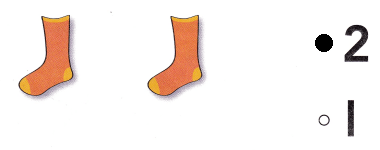# Texas Go Math Kindergarten Lesson 1.2 Answer Key Count and Write 1 and 2

Refer to our Texas Go Math Kindergarten Answer Key Pdf to score good marks in the exams. Test yourself by practicing the problems from Texas Go Math Kindergarten Lesson 1.2 Answer Key Count and Write 1 and 2.

## Texas Go Math Kindergarten Lesson 1.2 Answer Key Count and Write 1 and 2

Explore

DIRECTIONS: Count the cubes. Tell how many. Trace and write the numbers and words.Explanation:
Traced the number and the number name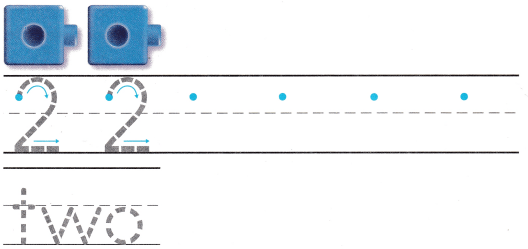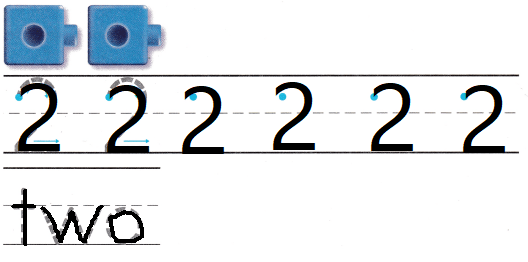Explanation:
Traced the number and the number name

Share and Show

DIRECTIONS: 1-2. Count the cubes. Soy the number. Practice writing the number. 3-4. Count and tell how many. Write the number.

Question 1.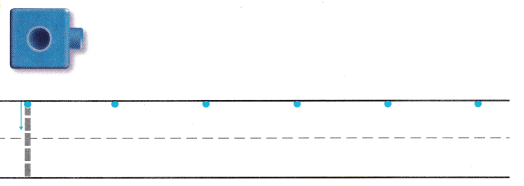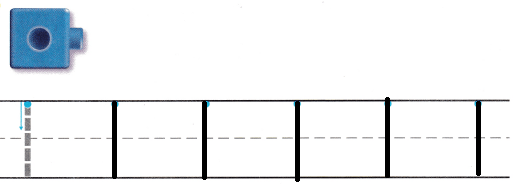Explanation:
Traced the number one

Question 2.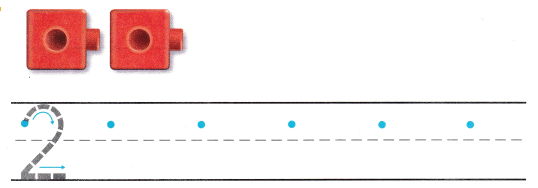Explanation:
Traced the number two

Question 3.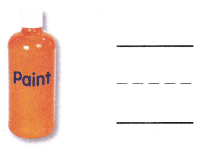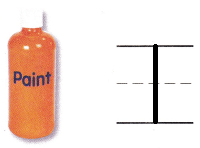Explanation:
Counted and Traced the number one

Question 4.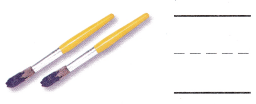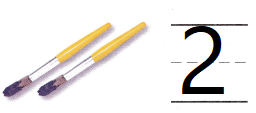Explanation:
Counted and Traced the number two

DIRECTIONS: 5-10. Count and tell how many. Write the number.

Question 5.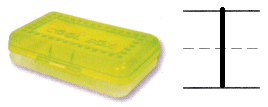Explanation:
Counted and Traced the number one

Question 6.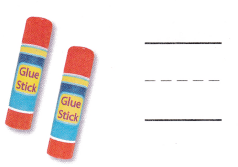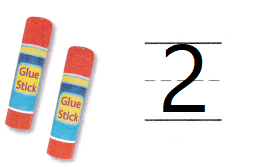Explanation:
Counted and Traced the number two

Question 7.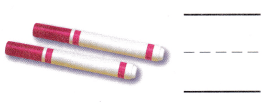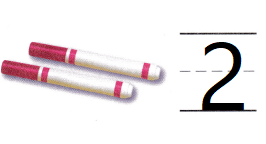Explanation:
Counted and Traced the number two

Question 8.Explanation:
Counted and Traced the number one

Question 9.Explanation:
Counted and Traced the number one

Question 10.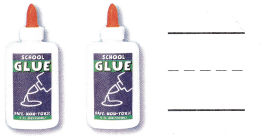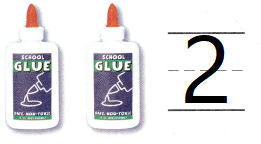Explanation:
Counted and Traced the number two

HOME ACTIVITY • Ask your child to write the number I on a paper. Then have him or her find an object that represents that number. Repeat with objects for the number 2.Explanation:
Activity to find the object relevant to the number has done.

DIRECTIONS: 11. Draw to show what you know about the number 1 or 2. Write the number beside your drawing. 12. Choose the correct answer. How many rockets are there?

Problem Solving

Question 11.Explanation:
Drawn and shown the number.

Question 12.Explanation:

### Texas Go Math Kindergarten Lesson 1.2 Homework and Practice Answer Key

DIRECTIONS: 1-6. Count and tell how many. Write the number.

Question 1.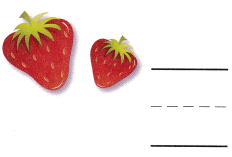Explanation:
Counted and traced the number 2

Question 2.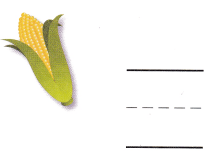Explanation:
Counted and traced the number 1

Question 3.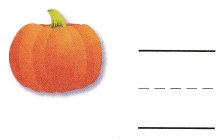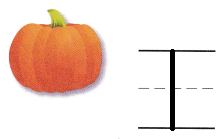Explanation:
Counted and traced the number 1

Question 4.Explanation:
Counted and traced the number 2

Question 5.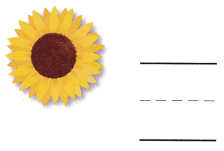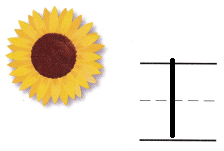Explanation:
Counted and traced the number 1

Question 6.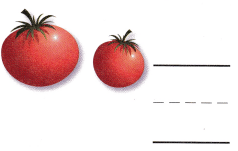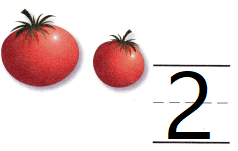Explanation:
Counted and traced the number 2

DIRECTIONS: Choose the correct answer. 7. How many hats are there? 8. How many shoes are there? 9. How many socks are there?

Lesson Check

Question 7.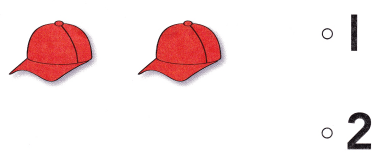Explanation:
Counted and bubbled the correct number
that is 2

Question 8.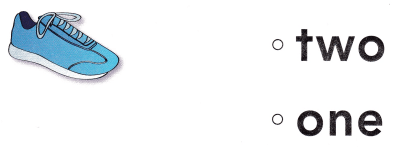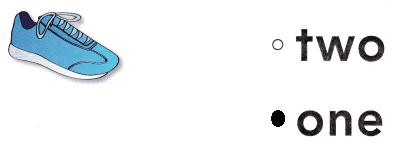Explanation:
Counted and bubbled the correct number
that is 1

Question 9.